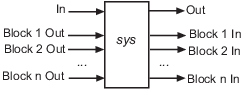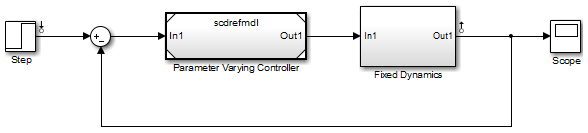# linlft

Linearize model while removing contribution of specified blocks

## Syntax

```lin_fixed = linlft(sys,io,blocks) [lin_fixed,lin_blocks] = linlft(___) [___] = linlft(___,opt) ```

## Description

`lin_fixed = linlft(sys,io,blocks)` linearizes the Simulink® model named `sys` while removing the contribution of certain blocks. Specify `sys` as a character vector or string. Specify the full block path of the blocks to ignore in the cell array of character vectors or string array called `blocks`. The linearization occurs at the operating point specified in the Simulink model, which includes the ignored blocks. You can optionally specify linearization points (linear analysis points) in the I/O object `io`. The resulting linear model `lin_fixed` has this form:The top channels In and Out correspond to the linearization points you specify in the I/O object `io`. The remaining channels correspond to the connection to the ignored blocks.

When you use `linlft` and specify the `'block-by-block'` linearization algorithm in `linearizeOptions`, you can use all the variations of the input arguments for `linearize`.

You can linearize the ignored blocks separately using `linearize`, and then combine the linearization results using `linlftfold`.

`[lin_fixed,lin_blocks] = linlft(___)` returns the linearizations for each of the blocks specified in `blocks`. If `blocks` contains a single block path, `lin_blocks` is a single state-space (`ss`) model. If `blocks` is an array identifying multiple blocks, `lin_blocks` is a cell array of state-space models. The full block path for each block in `lin_blocks` is stored in the `Notes` property of the state-space model.

`[___] = linlft(___,opt)` uses additional linearization options, specified as a `linearizeOptions` option set.

## Examples

collapse all

In this example, the `scdtopmdl` model contains two subsystems in the feedforward path.

• Fixed portion, which contains everything except the Parameter Varying Controller model reference

• Parameter Varying Controller model, which references the `scdrefmdl` model

Open the top-level model.

```topmdl = 'scdtopmdl'; open_system(topmdl)```Linearize this model without the Parameter Varying Controller block.

```io = getlinio(topmdl); blocks = {'scdtopmdl/Parameter Varying Controller'}; sys_fixed = linlft(topmdl,io,blocks);```

Linearize the controller model.

```refmdl = 'scdrefmdl'; load_system(refmdl); sys_pv = linearize(refmdl);```

Combine the linearization results.

`BlockSubs(1) = struct('Name',blocks{1},'Value',sys_pv);`

## Version History

Introduced in R2009b